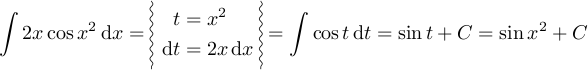# 2011-02-24 Wavy lines as delimiters (en)

Well, it’s been a while since I posted here; but today, I’ve got another real-life problem solution.

In Poland, it is customary to use “wavy lines” as delimiters when writing down integration by substitution or by parts. (This description is rather vague, I admit, so below is an example.)The problem is: how to obtain such an effect in LaTeX?

The problem is, surprisingly, not so easy. There is the MnSymbol font & package, which contains “wavy variable-size delimiters”, but it replaces all other math fonts with variants someone might not like (and is incompatible with amssymb/amsfonts).

Of course, tikz comes to rescue. Here is the code I used to obtain the above example:

\documentclass{article}

\usepackage{amsmath}

\usepackage{tikz}
\usetikzlibrary{decorations.pathmorphing}
\newcommand{\wavylrbox}{%
\begin{tikzpicture}[baseline=-0.5ex,decoration={snake,amplitude=0.75pt,segment length=5pt}]
\node (b) {$\displaystyle #1$};
\draw[decorate] (b.north west) -- (b.south west);
\draw[decorate] (b.north east) -- (b.south east);
\end{tikzpicture}
}

\begin{document}

\begin{equation*}
\int 2x\cos x^2\,\textup{d}x=\wavylrbox{
\begin{aligned}
t&=x^2\\
\textup{d}t&=2x\,\textup{d}x\\
\end{aligned}
}=\int \cos t\,\textup{d}t=\sin t+C=\sin x^2+C
\end{equation*}

\end{document}


(The \wavylrbox macro takes one argument and sets it in display math style surrounded from left and right—hence its name—by wavy lines.)

Unfortunately, this quick solution has a few problems.

1. If the height of the formula in “wavy lines” is not an integer multiple of 5pt, the waves have short straight-line segments at the bottom.
2. We assume here that the “central line” of the formula is at the height of 0.5ex. This might not be true in some fonts.
3. If the “wavy box” has an odd number of lines, it would be much better if the middle line were at the height of the rest of the formula. This seems to be tricky to obtain—most probably, instead of aligned or friends, one would have to use tikz’s matrices or something like that.

Still, tikz (as usually) does the trick nicely.

I’d be more than happy to see other/better solutions, especially ones that don’t have the above disadvantages!